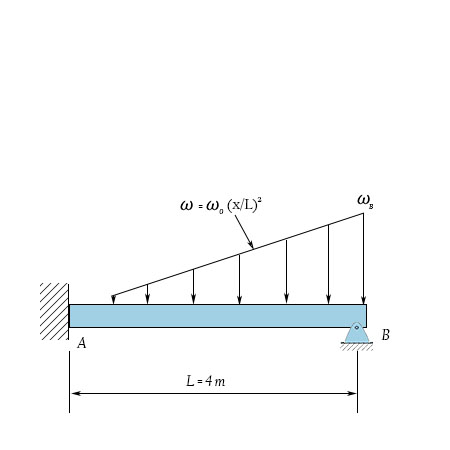# For the beam shown, determine the reaction at the roller support when \omega_0 = 16 kN/m. ...

## Question:

For the beam shown, determine the reaction at the roller support when {eq}\omega_0 {/eq} = 16 kN/m.## Reaction force

The force which acts at the support of a beam is termed as a reaction force. The reaction force acts in the opposite direction of the action force. In a simply supported beam, the reaction force acts at the roller end is only in one direction whereas the reaction force acts at the fixed support in x and y-direction both.

Given data:

• The distributed load acting on the beam is: {eq}{w_0} = 16\;{\rm{kN/m}} {/eq}.
• The function of the load on the beam is: {eq}w =...

Become a Study.com member to unlock this answer! Create your account